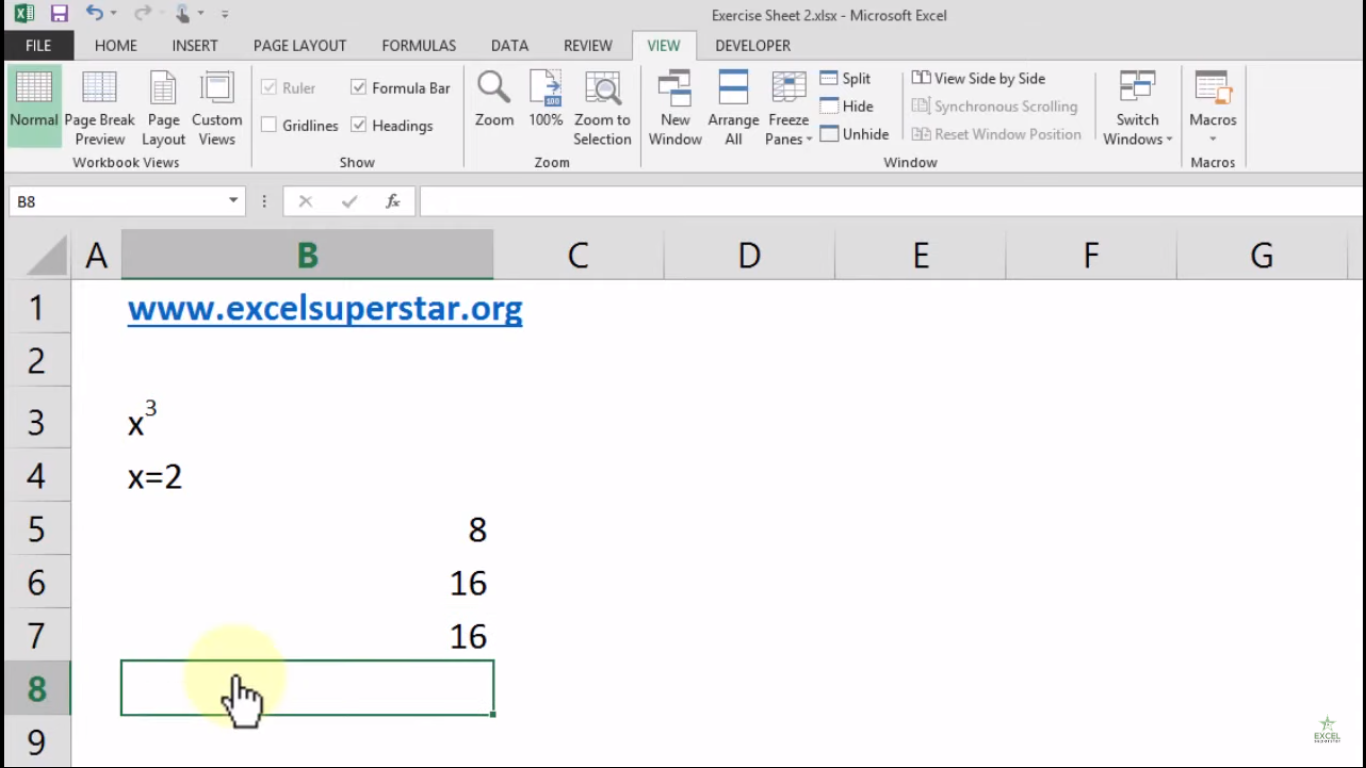# Excel Calculating Fixed Deposit maturity amountIn this tutorial, we will learn about Calculating Fixed Deposit maturity amount in Excel

Example: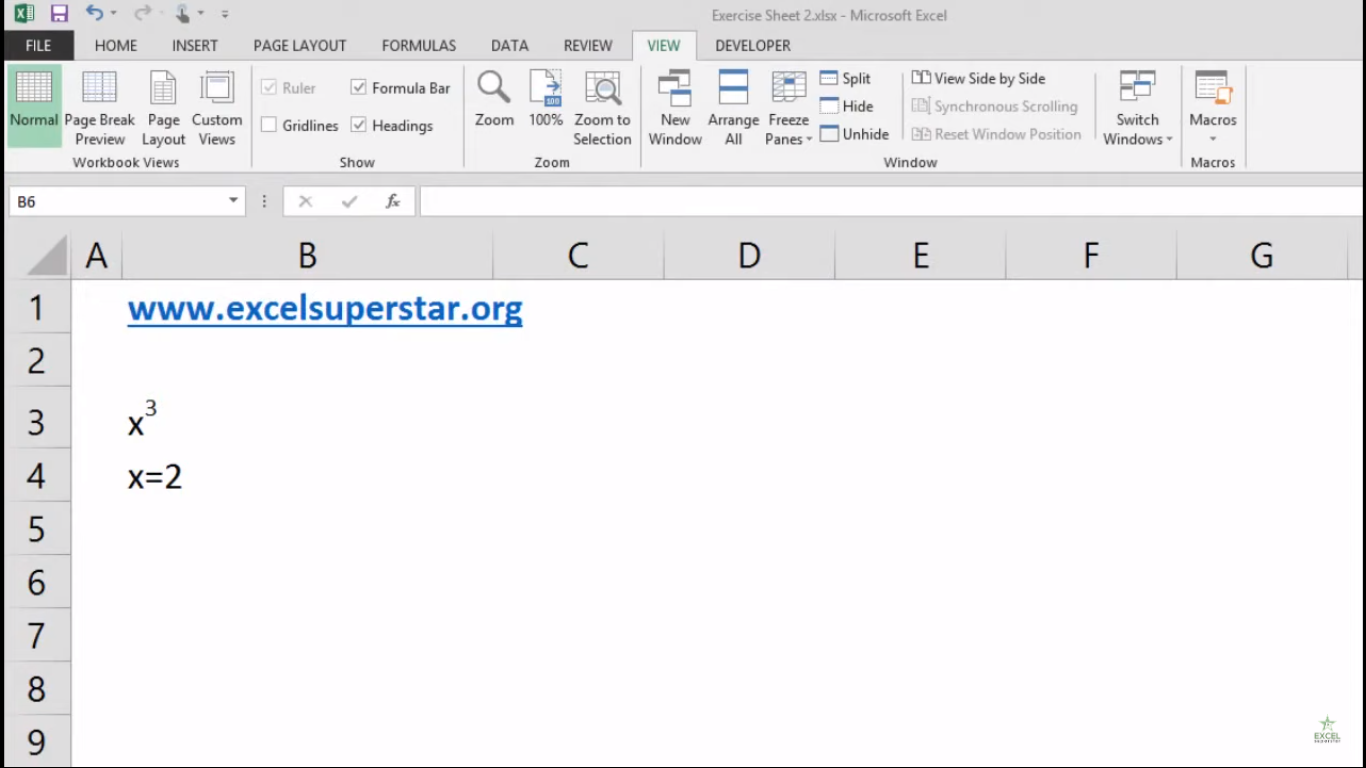I have an equation X3 and the value of X is equal to 2. Now I want to calculate it to get the answer. So to calculate the answer we have 2 different methods

1. Applying To the Power Sign

2. Applying Power Function

1. Applying To the Power Sign

1. Write =2^3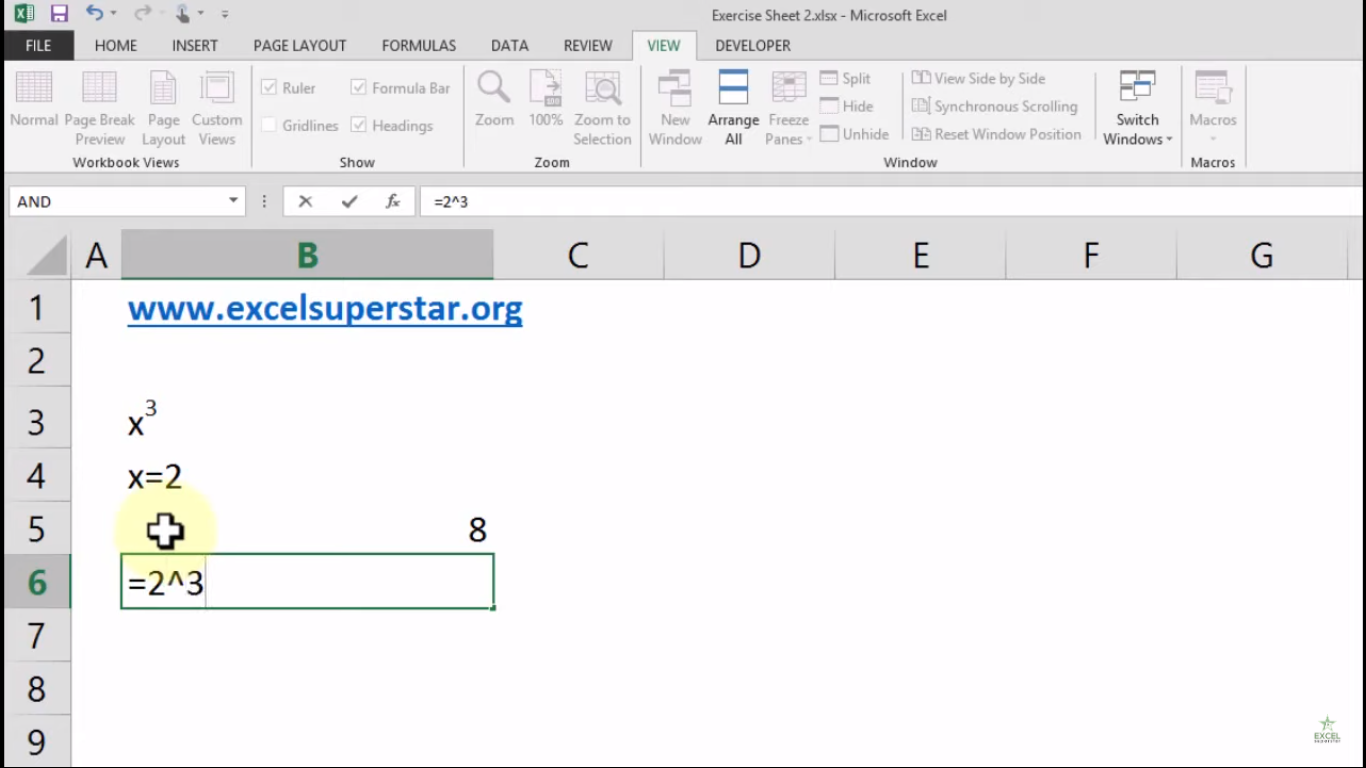Note – To the power sign is situated on the 6 digit number on your keyboard

2. Press Enter to get the answer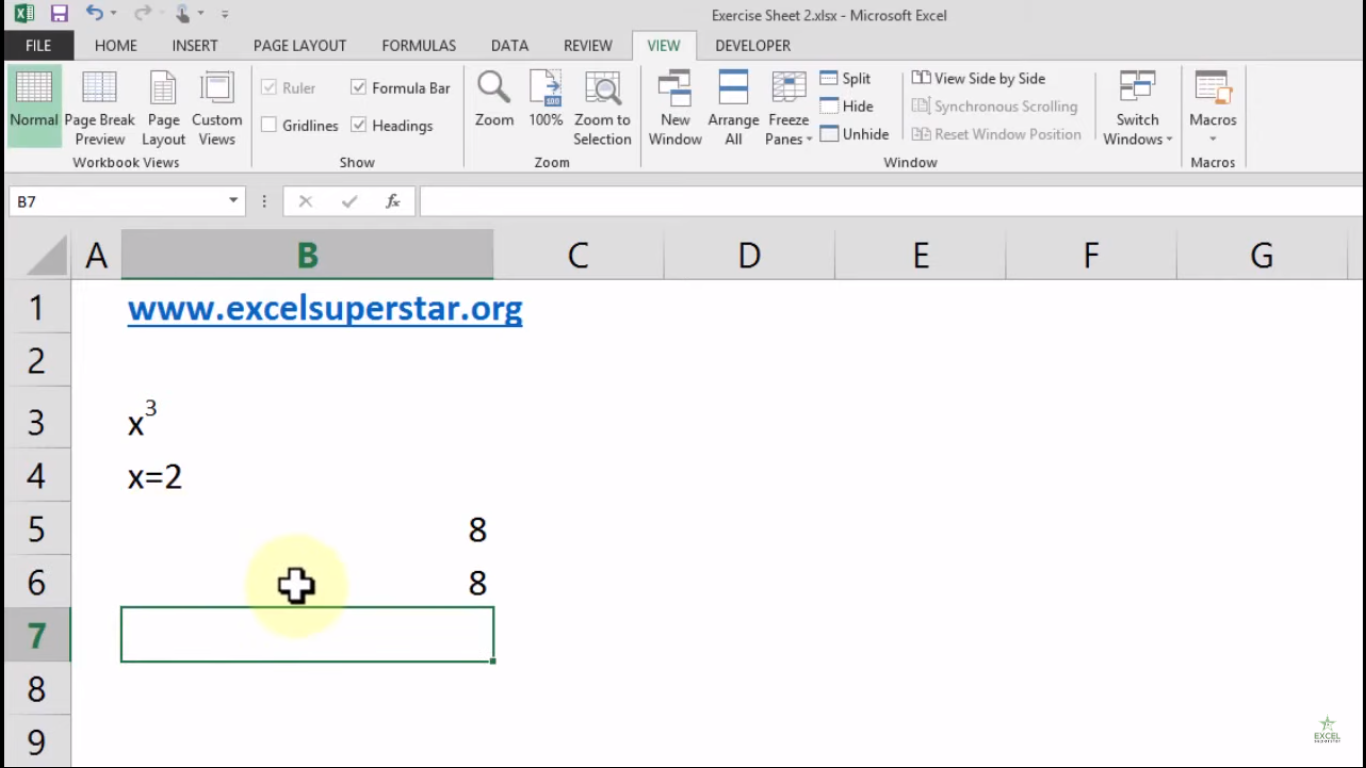2. Applying Power Function

The POWER function syntax has the following arguments:

1. Write =power and press the Tab Key1. Number – Number to raise to a power.

2. Power – Exponent to raise power to.

2. Write 2 as a number to raise power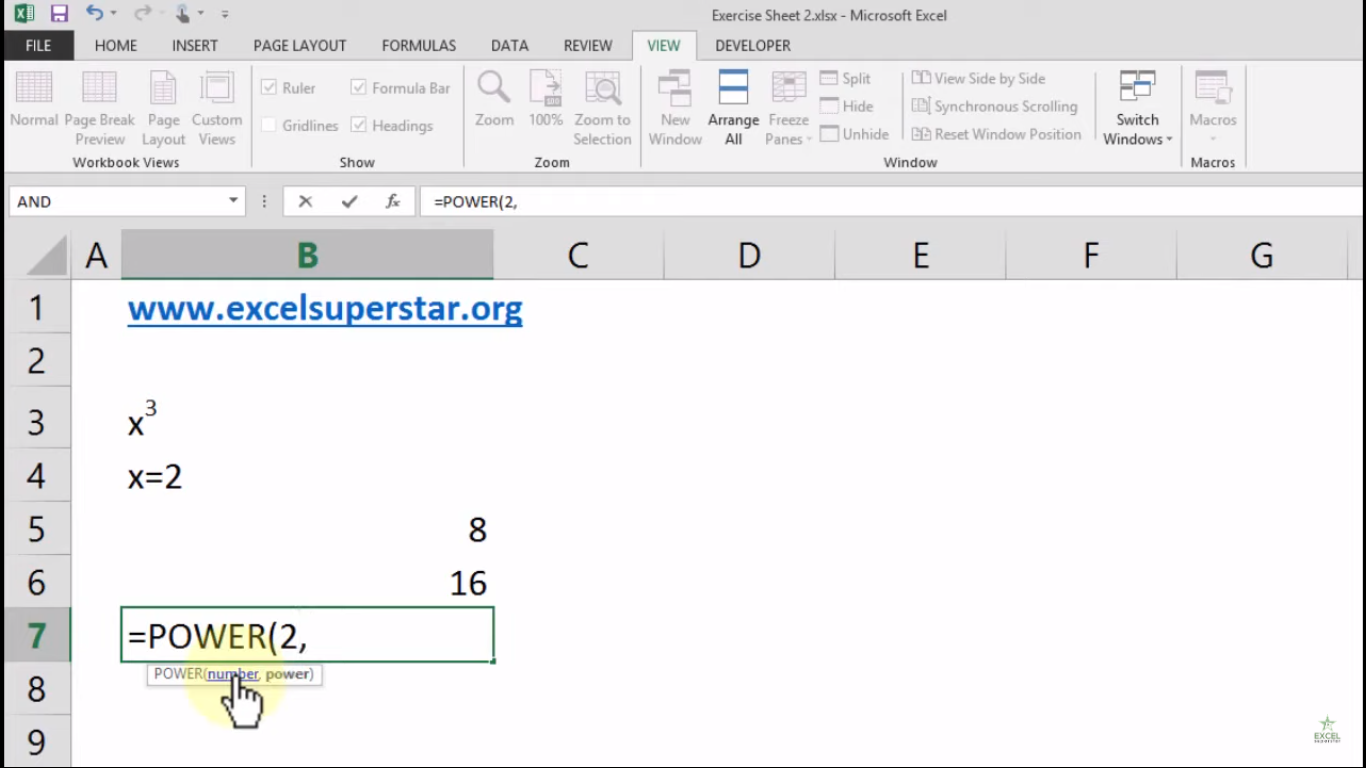3. Write 4 as an exponent to raise power to and Close the bracket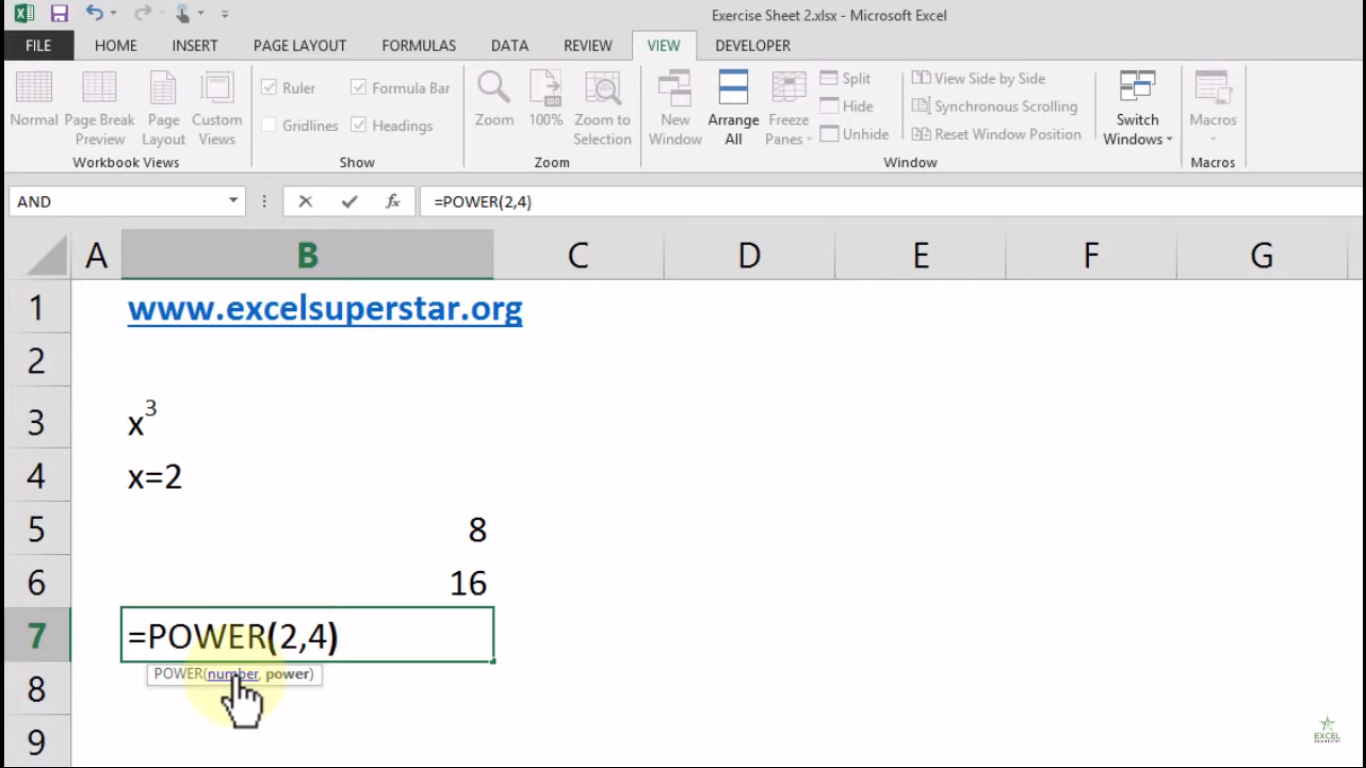4. Press Enter and Excel will calculate the answer